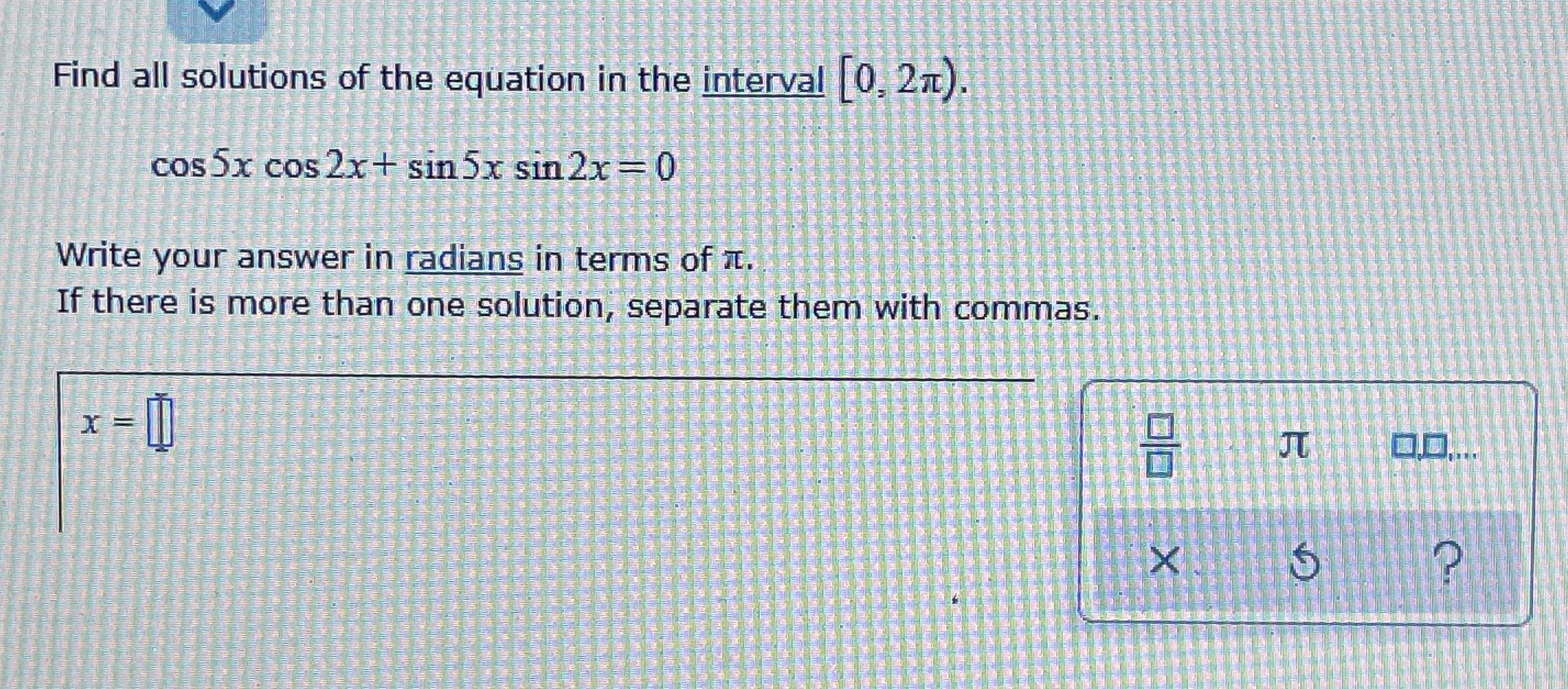### Still have math questions?

Algebra
QuestionFind all solutions of the equation in the interval $$[ 0,2 \pi )$$

$$\cos 5 x \cos 2 x + \sin 5 x \sin 2 x = 0$$

Write your answer in radians in terms of $$\pi$$ . If there is more than one solution, separate them with commas.

x=$$\frac{pi}{6}$$ x=$$\frac{pi}{2}$$ x=$$\frac{5pi}{6}$$ x=$$\frac{7pi}{6}$$ x=$$\frac{3pi}{2}$$ x=$$\frac{11pi}{6}$$